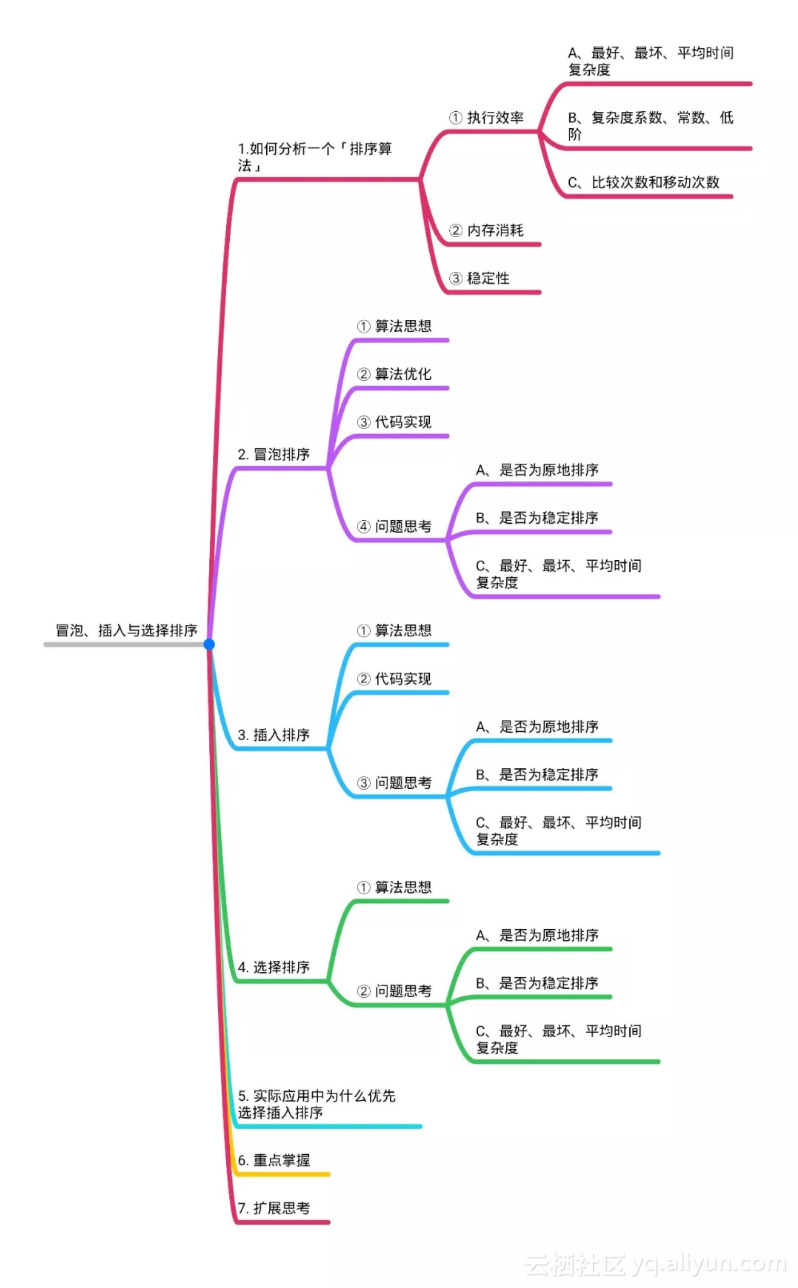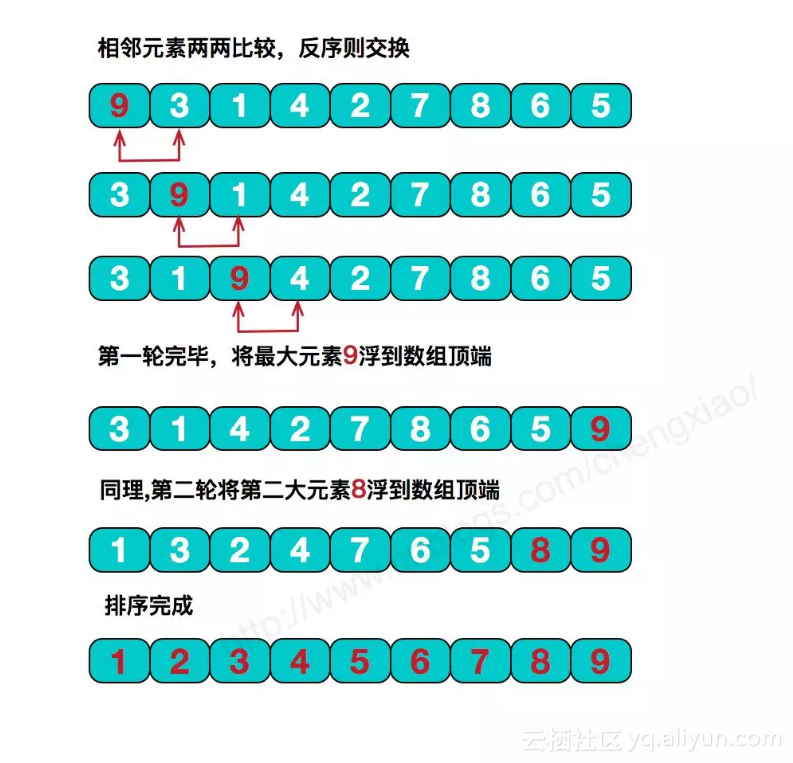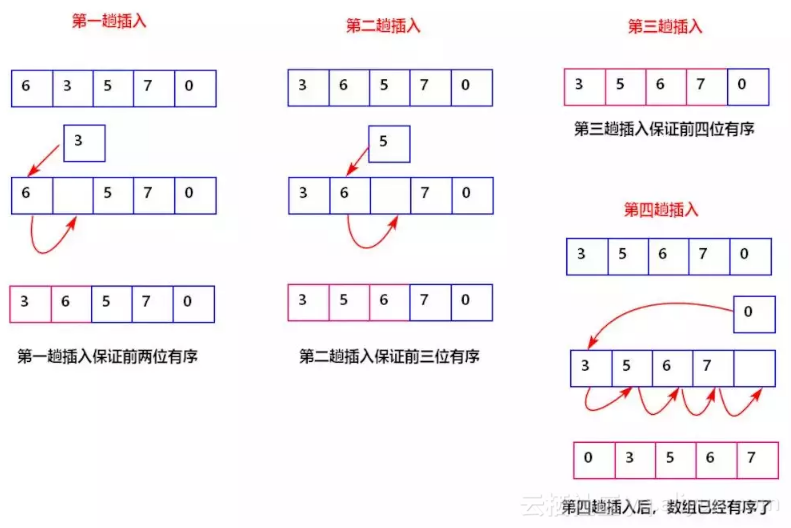面试前你必须知道的三个排序算法

+关注继续查看1. 执行效率

① 最好、最坏、平均时间复杂度

② 复杂度系数、常数、低阶

③ 比较次数和移动次数

2. 内存消耗

3. 稳定性

1、算法思想2、算法优化

3、代码实现

1// 冒泡排序，a 表示数组，n 表示数组大小
2public void bubbleSort(int[] a, int n) {
3 if (n <= 1) return;
4
5 for (int i = 0; i < n; ++i) {
6 // 提前退出冒泡循环的标志位
7 boolean flag = false;
8 for (int j = 0; j < n - i - 1; ++j) {
9 if (a[j] > a[j+1]) { // 交换
10 int tmp = a[j];
11 a[j] = a[j+1];
12 a[j+1] = tmp;
13 flag = true; // 表示有数据交换
14 }
15 }
16 if (!flag) break; // 没有数据交换，提前退出
17 }
18}

4、问题思考

①是否为原地排序

②是否为稳定排序

③最好、最坏以及平均时间复杂度

1、算法思想2、代码实现

1// 插入排序，a 表示数组，n 表示数组大小（从小到大进行排序）
2public void insertionSort(int[] a, int n) {
3 //如果数组大小为 1 直接返回
4 if (n <= 1) return;
5 //否则进行插入排序
6 for (int i = 1; i < n; ++i) {
7 int value = a[i];
8 int j = i - 1;
9 // 查找插入的位置
10 for (; j >= 0; --j) {
11 if (a[j] > value) {
12 a[j+1] = a[j]; // 数据移动
13 } else {
14 break;
15 }
16 }
17 a[j+1] = value; // 插入数据
18 }
19}

3、问题思考

① 是否为原地排序？

② 是否为稳定排序？

③ 最好、最坏、平均时间复杂度？

1、算法思想

2、问题思考

① 是否为原地排序

② 是否为稳定排序

③ 最好、最坏以及平均时间复杂度

3、代码实现

1// 选择排序，a表示数组，n表示数组大小
2 public static void selectionSort(int[] a, int n) {
3 if (n <= 1) return;
4 for (int i = 0; i < n; ++i) {
5 // 查找最小值
6 int minIndex = i;
7 int minValue = a[i];
8 for (int j = i; j < n; ++j) {
9 if (a[j] < minValue) {
10 minValue = a[j];
11 for (int i = 0; i < n - 1; ++i) {
12 // 查找最小值
13 int minIndex = i;
14 for (int j = i + 1; j < n; ++j) {
15 if (a[j] < a[minIndex]) {
16 minIndex = j;
17 }
18 }
19 if (minIndex == i)
20 continue;
21 // 交换
22 int tmp = a[i];
23 a[i] = a[minIndex];
24 a[minIndex] = tmp;
25 }
26 }

1冒泡排序中数据的交换操作：
2if (a[j] > a[j+1]) { // 交换
3 int tmp = a[j];
4 a[j] = a[j+1];
5 a[j+1] = tmp;
6 flag = true;
7}
8
9插入排序中数据的移动操作：
10if (a[j] > value) {
11 a[j+1] = a[j]; // 数据移动
12} else {
13 break;
14}10003 0909 0RSA与AES混合加密算法的实现
RSA与AES加密算法所产生的密钥数不一样，它们是如何进行加密的呢？ 接收方生成RSA密钥对，将其中的RSA公钥传递给发送方(接收方与发送方建立连接是需要认证的，SSL/TLS协议可以确保RSA公钥的安全完整)，然后用RSA公钥对AES密钥进行加密，加密后的结果传递给接收方，接收方用RSA私钥解密后，得到AES密钥，最后使用AES密钥解密，从而达到安全互通数据的目的。(如下图所示)
1569 010880 0RANSAC算法在图像拼接上的应用的实现

2290 013798 011879 0Silverlight中非对称加密及数字签名RSA算法的实现
RSA算法是第一个既能用于数据加密也能用于数字签名的算法。它易于理解和操作，也很流行。它的安全性是基于大整数素因子分解的困难性，而大整数因子分解问题是数学上的著名难题，至今没有有效的方法予以解决，因此可以确保RSA算法的安全性。
669 01185 07327 0

7208

9

《零基础CSS入门教程》

《零基础HTML入门教程》

《2021云上架构与运维峰会演讲合集》